## 67) Missing Number Equations

Bonds of 11 to 20: Racing Motorcycles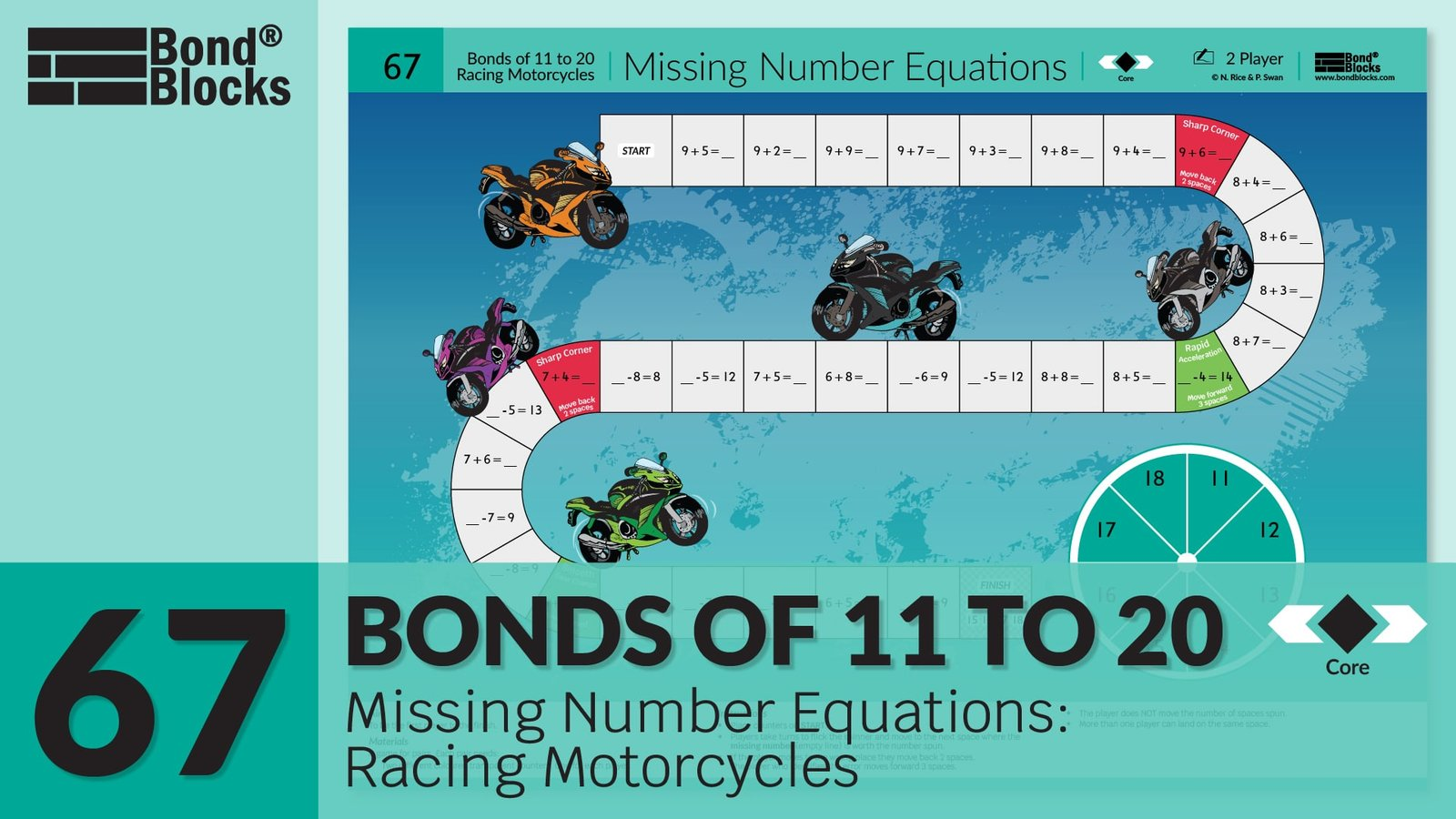### Mathematics

Develop fluency recalling single-digit, two-part bonds of 11, 12, 13, 14, 15,16 and 17 applied to addition and subtraction where the whole is the unknown.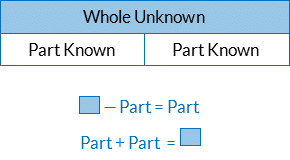The unknown whole in a subtraction equation is found by adding the known parts.### Language

• part-part-whole: where the two parts are a bond of the whole number
• known part
•  missing (unknown) whole
• subtract
• equals## Differentiation

### A little easier

##### Use Bond Blocks

Use Bond Blocks to to represent both parts and the whole.

For example,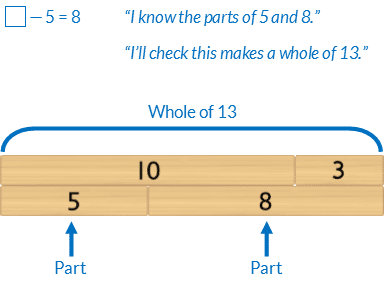If students cannot recall the bond encourage them to use what they know to work out what they don’t. Helpful strategies include:

• doubles and near doubles• bridging ten• partitioning using five plus bonds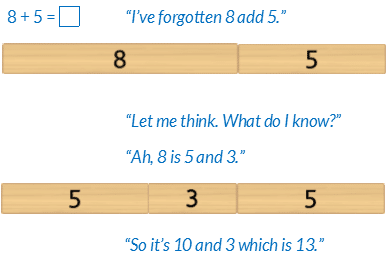##### Fluency cards

Each of the single-digit, two-part bonds for wholes 11 to 18 can be downloaded and printed as Addition and Subtraction Cards. Select cards for a specified bond/s and use these to play Memory, Go Fish, Snap where the goal is to collect related addition and subtraction equations for each whole.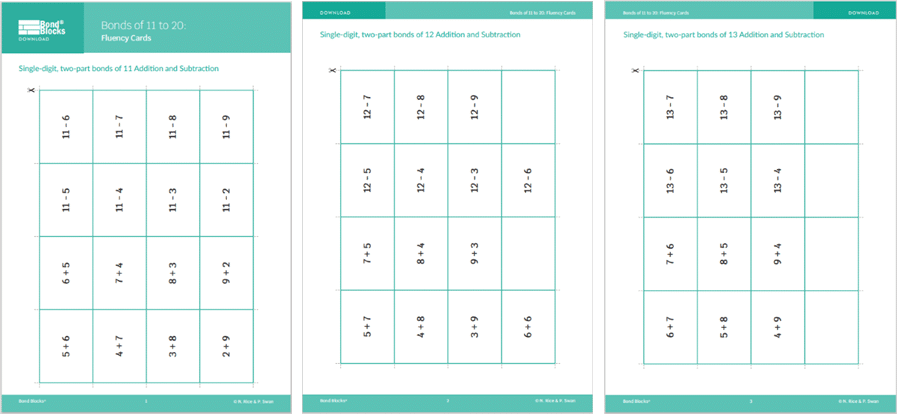### Progression

In the next activity, students apply concepts of addition and subtraction, and fluency recalling bonds of wholes up to 20, to solve word problems. Go to

##### Activity 68

Bonds of 11 to 20: Word Problems, Wholes to 20